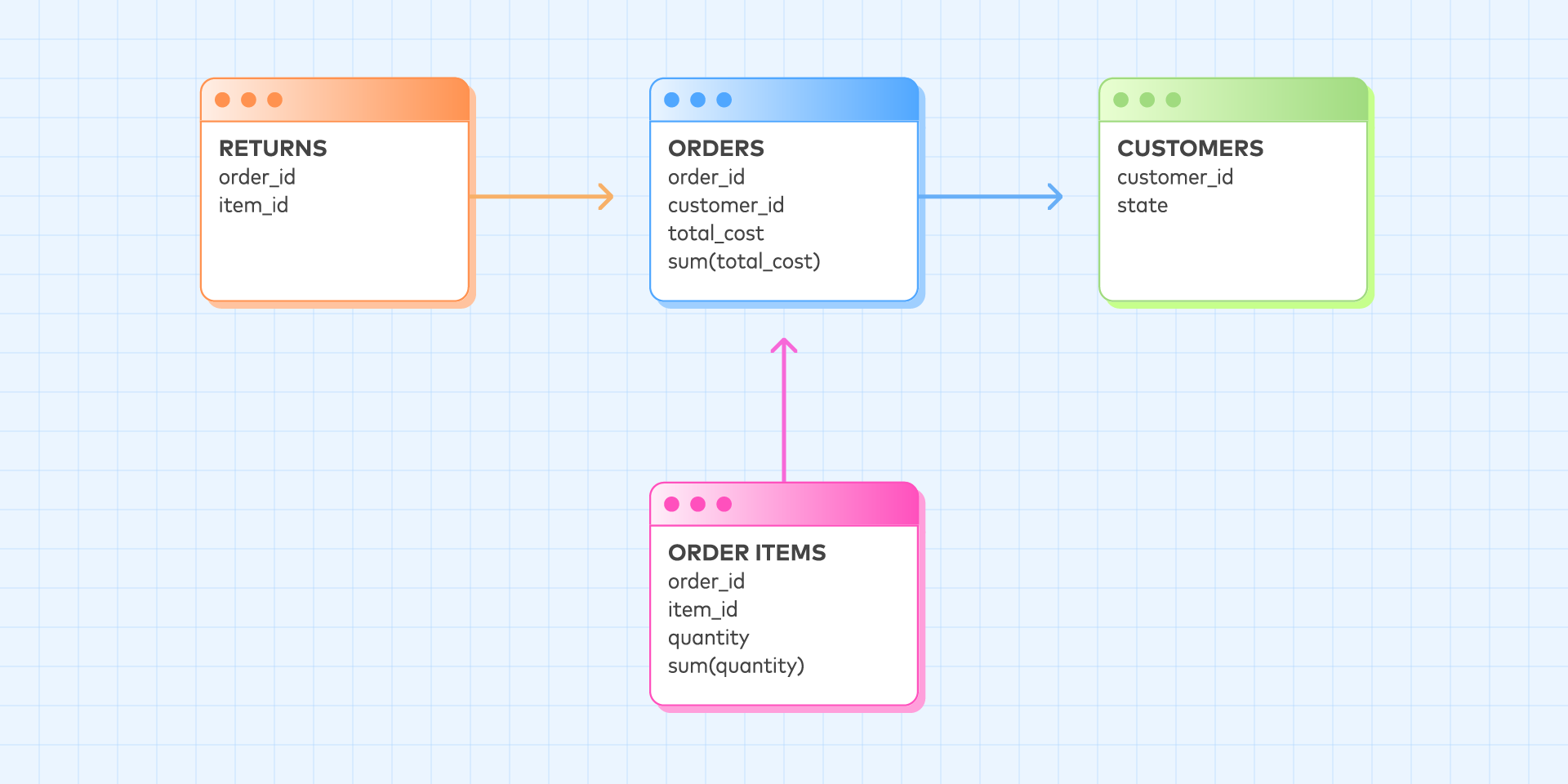# How to Use Measures With One-to-Many Joins

Make sure you don’t duplicate aggregated values when you join tables together."How can I implement measures with one-to-many joins?" is a classic problem in dimensional modeling. Let's consider a simple example. Suppose we work with the dimensional model in the image above.

There are two measures in this model: sum(orders.total_cost) and sum(order_items.quantity). The "fact table" is orders, but we're using the term "fact table" loosely because orders has one-to-many relationships with order_items and returns. Suppose we want to answer the question:

• For each state…
• ...what is the total cost of all orders?
• ...and the total number of ordered items?

Put another way, we would like to construct the following pivot table:

state sum(total_cost) sum(quantity)
Alabama ? ?
... ... ...

Naively, we might use the following SQL query to answer this question:

select   state,   sum(total_cost),   sum(quantity) from orders join order_items using (order_id) join customer using (customer_id) group by 1

However, this SQL query would produce a result that violates our intuition about the meaning of these measures. To understand why, suppose our tables are populated with the following data:

ORDERS

order_id customer_id total_cost
1 11 \$1
2 12 \$10

ORDER_ITEMS

order_id item_id quantity
1 apple 1
2 apple 2
2 orange 1

CUSTOMERS

customer_id state
11 Alabama

The result of our naive SQL query would be:

state sum(total_cost) sum(quantity)
Alabama \$1 1

The sum(total_cost) for Alaska is \$20, despite the fact that there was only one order in Alaska with a total cost of \$10. This occurs because of the one-to-many join from orders to order_items; this single order was counted twice because it had two items in it.

Looker solves this problem using a clever trick called symmetric aggregates. (It is so clever they have patented it.) Looker would generate a query of the form:

select state, sum(distinct hash(order_id) + total_cost) - sum(distinct hash(order_id)), sum(quantity) from orders join order_items using (order_id) join customer using (customer_id) group by 1

The clever part is replacing sum(total_cost) with:

sum(distinct hash(order_id) + total_cost) - sum(distinct hash(order_id))

This funky-looking SQL ensures that each row of orders only contributes to the sum(total_cost) measure once. This approach works, but it has several disadvantages:

• It's complicated to write this SQL by hand.
• If you have multiple one-to-many joins, it can generate huge intermediate results.
• Distinct aggregates are slower than their simple counterparts.

If you're trying to accomplish the same thing with hand-written SQL, there is an alternative approach that solves these problems:

select state, sum(total_cost), sum(quantity) from orders join (     select order_id, sum(quantity) as quantity   from order_items     group by 1 ) using (order_id) join customers using (customer_id) group by 1

We have turned the one-to-many relationship into a one-to-one relationship by doing multiple levels of aggregation using a subquery. Subqueries have a bad reputation among analysts because older database systems execute them using inefficient recursive evaluation, but any modern data warehouse will generate an efficient plan from the above query.

As a bonus, we've avoided the use of more costly distinct aggregates, and there's no possibility of a "combinatorial explosion" producing huge intermediate results. To my knowledge, no BI tool currently uses this technique, but if you're writing SQL queries by hand, this is a useful trick.